##### Inorganic Chemistry For Dummies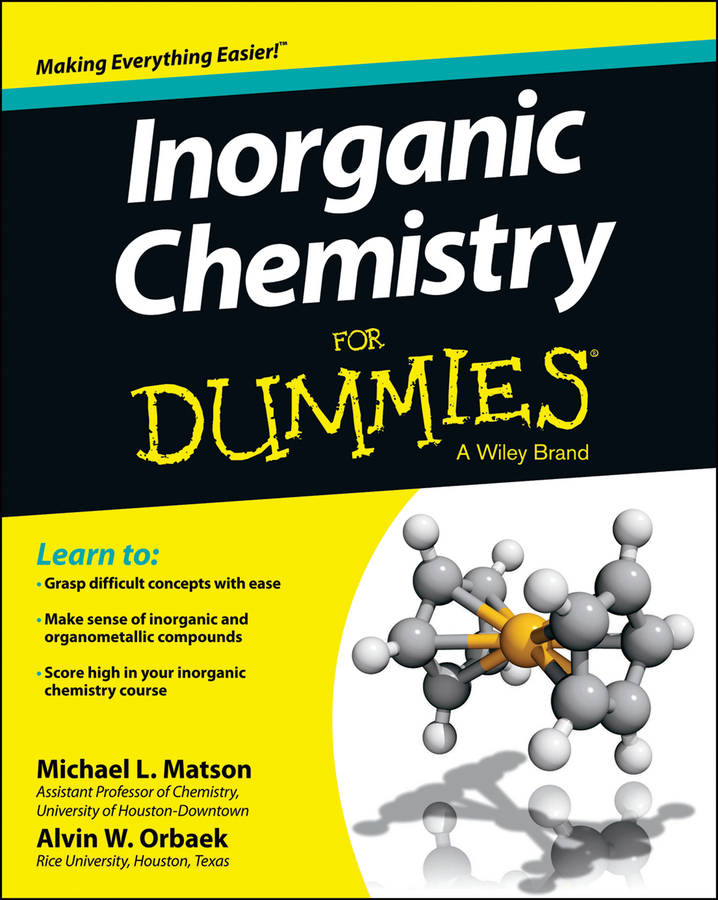When an ionic compound is formed, the cation and anion attract each other, resulting in a salt. An important thing to remember is that the compound must be neutral — that is, it has equal numbers of positive and negative charges.

## Putting magnesium and bromine together

Suppose you want to know the formula, or composition, of the compound that results from reacting magnesium with bromine. You start by putting the two atoms side by side, with the metal on the left, and then adding their charges. The following figure shows this process.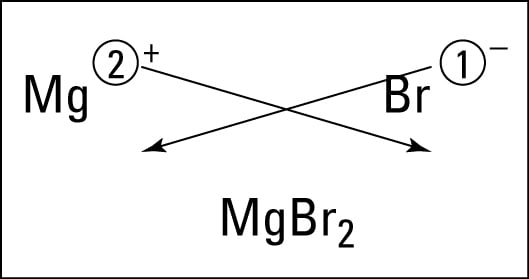Figuring the formula of magnesium bromide
The electron configurations for magnesium and bromine are: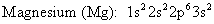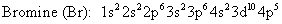Magnesium, an alkaline earth metal, has two valence electrons that it loses to form a cation with a 2+ charge. The electron configuration for the magnesium cation is: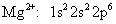Bromine, a halogen, has seven valence electrons, so it gains one to complete its octet (eight valence electrons) and form the bromide anion with a 1- charge. The electron configuration for the bromide anion is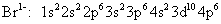The compound must be neutral; it must have the same number of positive and negative charges so that, overall, it has a zero charge. The magnesium ion has a 2+, so it requires 2 bromide anions, each with a single negative charge, to balance the 2 positive charges of magnesium. So the formula of the compound that results from reacting magnesium with bromine is: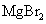## Using the crisscross rule

There’s a quick way to determine the formula of an ionic compound: Use the crisscross rule.

Look at the preceding figure for an example of using this rule:
1. Take the numerical value of the metal ion’s superscript (forget about the charge symbol) and move it to the bottom right-hand side of the nonmetal’s symbol — as a subscript.

2. Then take the numerical value of the nonmetal’s superscript and make it the subscript of the metal.

Note that if the numerical value is 1, it’s just understood and not shown.

So in this example, you make magnesium’s 2 a subscript of bromine and make bromine’s 1 a subscript of magnesium (but because it’s 1, you don’t show it), and you get the formula:So what happens if you react aluminum and oxygen? The figure below shows the crisscross rule used for this reaction.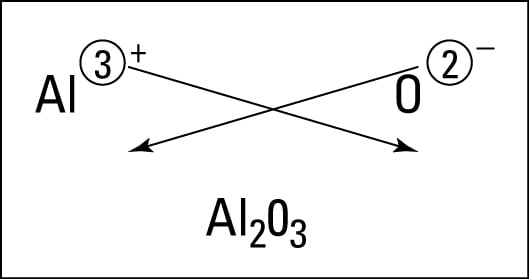Figuring the formula of aluminum oxide
Compounds involving polyatomic ions work exactly the same way. For example, here’s the compound made from the ammonium cation and the sulfide anion: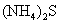Notice that because two ammonium ions (two positive charges) are needed to neutralize the two negative charges of the sulfide ion, the ammonium ion is enclosed in parentheses and a subscript 2 is added.

The crisscross rule works very well, but there’s a situation where you have to be careful. Suppose that you want to write the compound formed when magnesium reacts with oxygen. Magnesium, an alkaline earth metal, forms a 2+ cation, and oxygen forms a 2- anion. So you might predict that the formula is: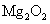But this formula is incorrect. After you use the crisscross rule, you need to reduce all the subscripts by a common factor, if possible. In this case, you divide each subscript by 2 and get the correct formula:

MgO